# Integration as the inverse of differentiation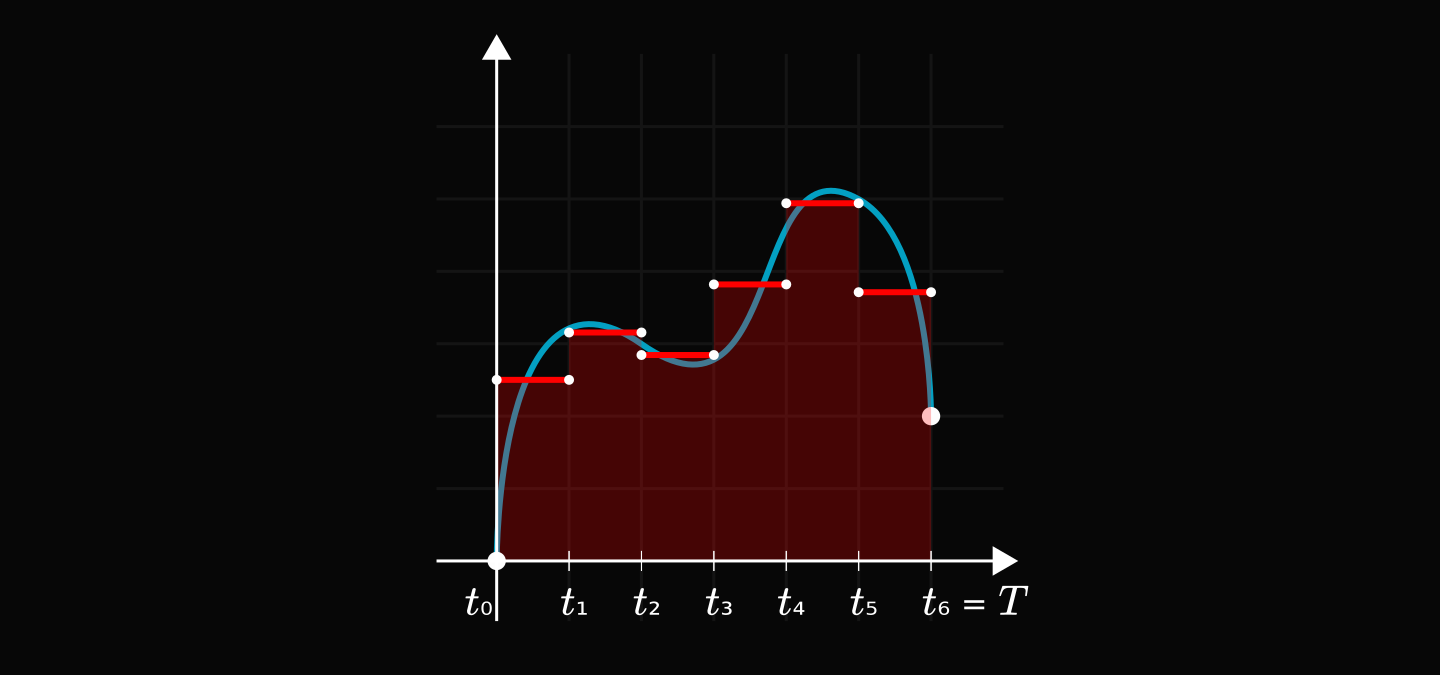One surprising fact about calculus: differentiation can be inverted!

An even more surprising fact: its inverse is integration.

After reading this short post, you'll be amazed by the brilliance of Newton's calculus.

## What is the derivative, really?

Let's start at the basics! Suppose that we have a moving object. For simplicity, let's assume that it moves along a straight line.

We can fully describe its dynamics with its time-distance function. For example, this is a constant velocity motion.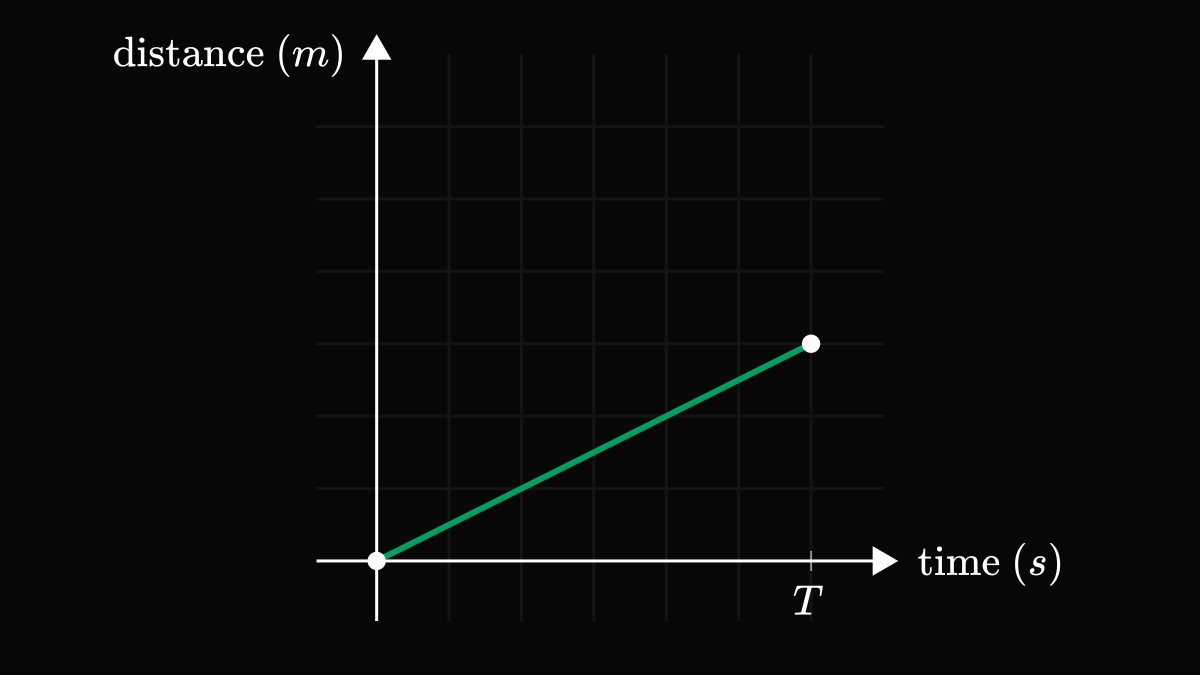As Newton discovered, the derivative of the time-distance function is its speed! In the case of a constant velocity motion, the speed is constant. (As properly reflected by its name.)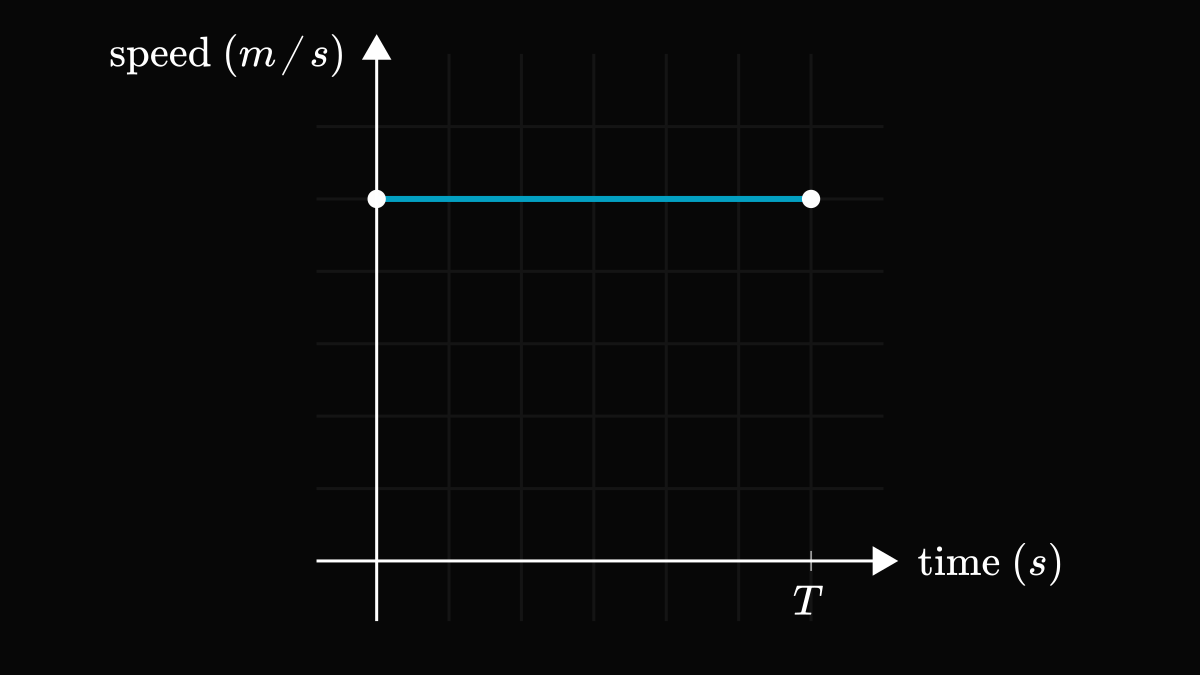To sum up, speed is the derivative of distance.

The question is if we only know the speed of our moving object, can we reconstruct the distance traveled?

## Reconstructing distance from speed

For our constant velocity motion, this is easy to do: if an object is moving at speed $v$ for time $T$, the total distance traveled is $v T$.This quantity also coincides with the area under the time-speed graph. Is this a coincidence? No, as we shall see next.

Does the area under the time-speed graph equal the distance traveled in the general case?

For instance, what happens when the time-speed plot looks something like this?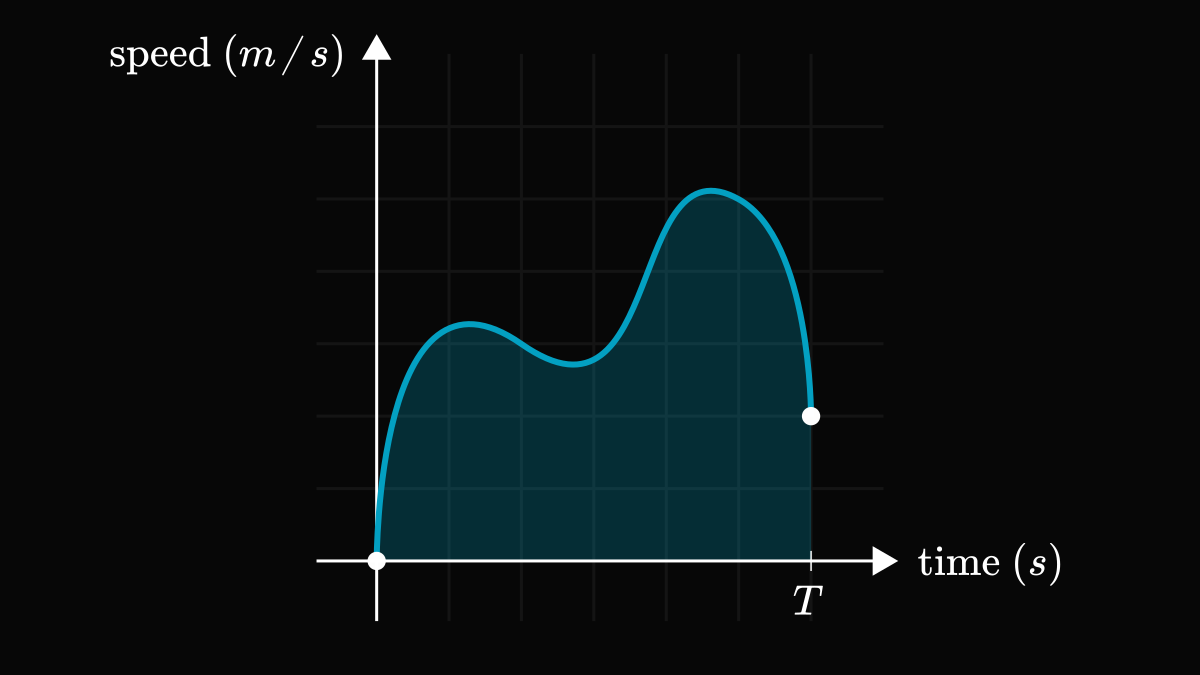In this case, we can do a simple trick: partition the time interval $[0, T]$ into smaller ones and approximate the object’s motion as a constant velocity motion on each of these intervals. Thus, we can reduce the general case to a special one that we have already seen.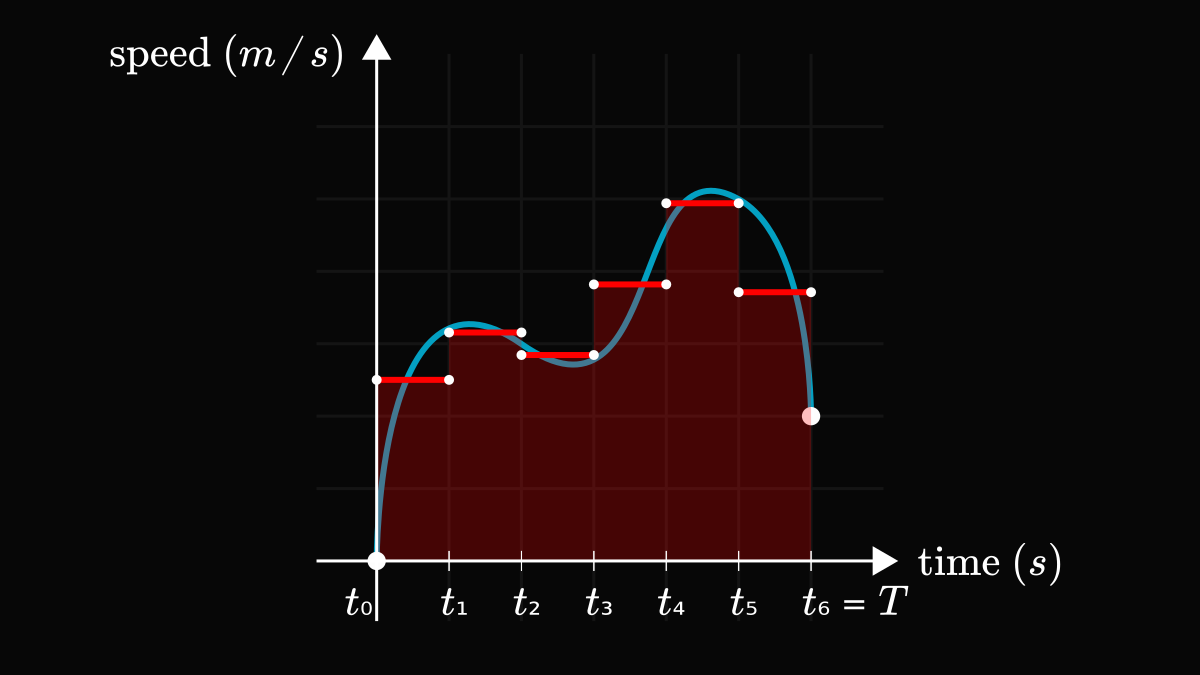If the time intervals are sufficiently granular, the distance traveled will roughly match a constant velocity motion with the average speed in a given interval.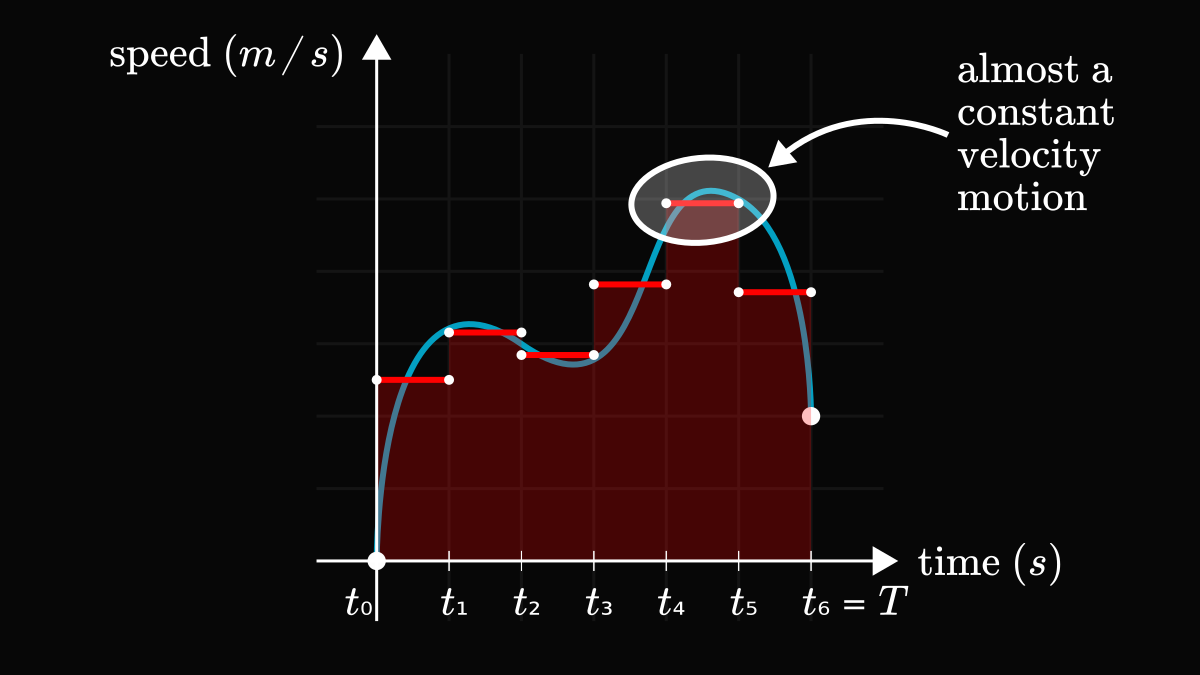In mathematical terms, we can approximate the total distance traveled with a finite sum:

\begin{align*} v_i := &\text{average speed in } [t_{i-1}, t_i], \quad i = 1, 2, \dots, n, \\ & \sum_{i=1}^{n} v_i (t_i - t_{i - 1}) \approx \text{ total distance}. \end{align*}

Notice that this sum approximates the area under the time-speed graph as well!

If we increase the granularity of the partition, this sum will converge to the true area under the curve:

$\sum_{i=1}^{n} v_i (t_i - t_{i - 1}) \to \int_{0}^{T} v(t) dt.$

This is what we call integration.

Notice that the integral represents the signed area, meaning that if the curve goes below the x-axis, the area is negative. This is not surprising since negative speed means backward movement.

To sum up once more, speed is the derivative of distance, and distance is the integral of speed. This is synthesized by the Newton-Leibniz formula, one of the pinnacle results in calculus:

$f(T) = f(0) + \int_{0}^{T} f^\prime(t) dt.$

This beautiful expression shows that integration is the inverse of differentiation. Next time you feel confused about the relation if integration and differentiation, just think about the Newton-Leibniz formula! It'll clear up that confusion in no time.